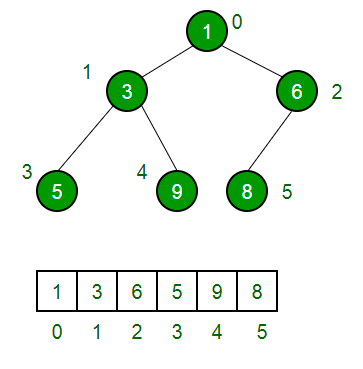# Leaf starting point in a Binary Heap data structure

Binary Heap is a complete tree (All levels are completely filled except possibly the last level and the last level has all keys as left as possible). In other words, we can say that it’s an almost complete binary tree.

A Binary heap is typically represented as array. If we take a closer look, we can noticed that in a Heap with number of nodes n, the leaves start from a particular index and following it, all the nodes are leaves till index n.

Let’s see an example to observe this:

```            10
/      \
20        100
/
30
```

Let us represent this in the form of an array Arr whose index starts from 1 :
we have:
Arr = 10
Arr = 20
Arr = 100
Arr = 30
If we observe, the first leaf (i.e. 100) starts from the index 3. Following it Arr is also a leaf.

By carefully analyzing, the following conclusion is observed:

The first leaf in a Heap starts from [floor(n/2)]+1 and all the nodes following it till
n are leaves.

Conclusion: In a Heap having n elements, Elements from indexes [(floor(n/2)+1) to n] are leaves.

What is starting index of leaves if indexes start from 0 instead of 1?
The above explanation assumes indexes starting from 1, but in most of the programming languages, index starts with 0.If we consider 0 as starting index, then leaves starts from floor(n/2) and exist till end, i.e., (n-1).

This article is contributed by Ranju Kumari. If you like GeeksforGeeks and would like to contribute, you can also write an article using contribute.geeksforgeeks.org or mail your article to contribute@geeksforgeeks.org. See your article appearing on the GeeksforGeeks main page and help other Geeks.